# Visualizing Seven-Manifolds

In 1956 John Milnor startled the mathematical community by constructing smooth seven-dimensional manifolds that are homeomorphic but not diffeomorphic to the standard seven-sphere. His discovery opened a new branch of research in topology and won him the Fields Medal in 1962.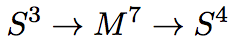The animation below gives a quick overview of the construction and shows a visualization of exotic seven-manifolds. An analogous structure in lower dimensions is shown in the Hopf fibration animation. If you have any questions or comments, I'd love to hear them!

## The animation

This animation was presented at the Second Abel Conference: A Mathematical Celebration of John Milnor, in January 2012.

You can play the video directly from this page, but it's worth viewing in a larger format directly from the YouTube player.

If you want, you can jump to the end of the slides at 3:10. You can also download a pdf copy of the introductory slides.

## Things to look for while you watch

• Under stereographic projection, the quaternion 1 maps to the boundary of the three-ball. Since this point is fixed by quaternion conjugation, the structure maps $$\xi_{h,-h}$$ look like 3-dimensional rotations. In fact they are 3-dimensional rotations because the real-axis of the quaternions is fixed by conjugation.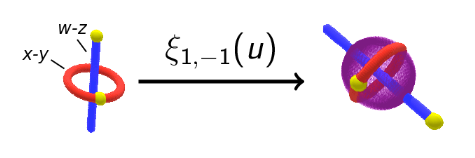• Multiplication by a unit quaternion (other than 1) has no fixed points on the three-sphere, so other structure maps do not preserve the boundary of the three ball.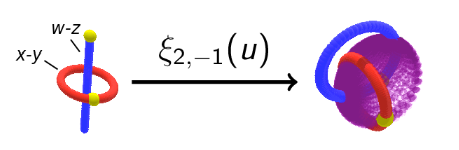• Under the structure map $$\xi_{1,0}(u)$$, the stereographic projection of the w-z reference circle always lies in the same plane as the projection of the Hopf fiber along which $$u$$ lies. That's why the shape formed by stacking these circles looks like a straight tube instead of a curly one.
• The difference between the structure maps of two seven speres is a structure map of the form $$\xi_{h, -h}$$. The positioning of reference curves for $$M(1,-1)$$, for example, explains how the tubes for $$S^7_1$$ and $$S^7_3$$ differ. This gives a way of imagining a non-smooth homeomorphism between the two different spheres.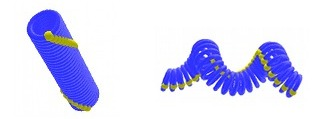• The spheres $$S^7_1$$ and $$S^7_{15}$$ are not distinguished by the invariant $$\lambda(S^7_k)$$. Are they diffeomorphic? This exercise for the viewer can be answered by using the A-hat genus. Later work of Kervaire-Milnor shows that there are 28 distinct diffeomorphism types of seven-spheres. They form the group $$\mathbb{Z}/28$$ under connected sum. Work of Eells-Kuiper completely describes the diffeomorphism types of seven-spheres which can appear as $$S^3$$ bundles over $$S^4$$.
• Many more details can be found on the Exotic spheres page of the Manifold Atlas Project.

## Acknowledgements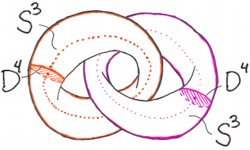Two copies of S^3 x D^4 glued along their boundary.

This project began when Doug Ravenel described some ideas for visualizing Milnor's constructions. I'm grateful for his feedback, and for discussions with several of the UGA topologists.

I would also like to thank Diarmuid Crowley for pointing out an error in a previous version of the introduction! Here is the correct statement: When $$h+j = 0$$, the total space of the bundle $$\xi_{h,-h}$$ is homeomorphic to $$S^3 \times S^4$$ if and only if $$h = 0$$. It is homotopy equivalent to $$S^3 \times S^4$$ if and only if $$h$$ is divisible by 12. In fact the total spaces of $$\xi_{0, 0}$$, $$\xi_{1,-1}$$ and $$\xi_{2,-2}$$ represent three distinct homotopy types with the same integral homology groups as $$S^3 \times S^4$$. The Euler classes of these bundles are trivial, but their first Pontrjagin classes are distinct: it follows that the total spaces are not homeomorphic. The homotopy, homeomorphism and diffeomorphism types of manifolds associated to $$\xi_{h,j}$$ were completely classified in work of Crowley and Escher: A classification of S^3-bundles over S^4, Differential Geometry and its Applications, 2003.

### Narration

Nemili Johnson performed the excellent narration; it is a pleasure to thank her for working with me.

### Computer Software

All of the software used for this animation is open-sourced and available on a variety of platforms.

The Python-based mathematics program Sage was used for determining the fiber parametrizations and keeping track of all the animation data. Sage provides an interface to the ray tracing system Tachyon, and this is what produced the individual frames.

The soundtrack is adapted from I'm Your Moon, by Jonathan Coulton. His music is released under a CC License, and the music was edited with Audacity. To stich the frames into an animation, I used FFmpeg, a full-featured program for working with audio and video in a broad range of codecs.

This project also depends on roughly 2000 lines of code which computes diffeomorphisms of the three-sphere and draws them at appropriate points in each frame. This is largely based on code written for the Hopf fibration animation, available under a GNU General Public License.

### Rendering:

The frames for this animation were rendered on the high-powered machines made available by Jonathan Hanke at the University of Georgia, and I'm extremely grateful for this support. Raytracing the final product took 40 modern processors about 3 days.

tags: animation | sage

## Site InfoThe content of nilesjohnson.net is licensed under CC BY-NC 4.0.